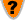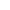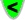What is the Zwicky Constant?

 Q.  What is the Zwicky Constant?A.  As light travels through the Universe's gravitational field, it slowly loses energy. The Zwicky Constant defines this loss.Q.  How is it defined?A.  The Zwicky Constant is the distance light travels to lose half its energy.Q.  How is it measured?A.  Spectral lines in the light from distant objects are shifted towards redder (lower-energy) wavelengths (red-shifts), and these red-shifts are greater, the greater the distance the light has travelled.Q.  What's the value of the Zwicky Constant?A.  About 7.7 billion light-years.Q.  Doesn't the Hubble Constant measure the same thing?A.  It's related, but the Hubble Constant is given as a rate of expansion of the Universe, using an incorrect model.Q.  Where is there more detail on this?